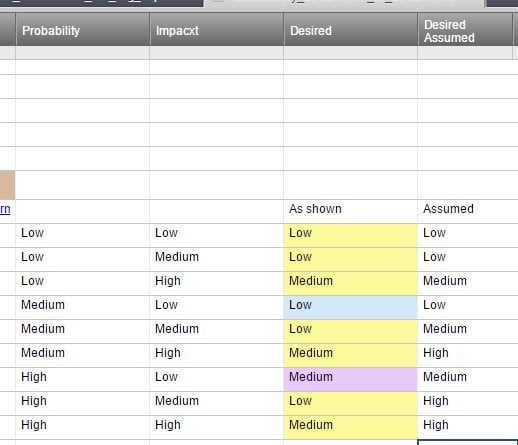#### Welcome to the Smartsheet Forum Archives

The posts in this forum are no longer monitored for accuracy and their content may no longer be current. If there's a discussion here that interests you and you'd like to find (or create) a more current version, please Visit the Current Forums.

# Nested IF/AND Formula

Hello

I am trying to create a matrix using the IF/AND Formula. So far the first part of the formula works which is this:

=IF(AND(Probability1 = "Low", Impact1 = "Low"), "Low", IF(Impact1 = "Medium", "Low", IF(Impact1 = "High", "Medium")

Now I want to add the other combinations

((Probability1 = "Medium", Impact1 = "Low"), "Low", IF(Impact1 = "Medium", "Medium", IF(Impact1 = "High", "High"))

also

((Probability1 = "High", Impact1 = "Low"), "Medium", IF(Impact1 = "Medium", "High", IF(Impact1 = "High", "High"))

How can I add these 2 remianing parts?

Tags:

• You need to combine them by adding them to you last open False option.  It will look something like this.

=IF(AND(Probability1 = "Low", Impact1 = "Low"), "Low", IF(Impact1 = "Medium", "Low", IF(Impact1 = "High", "Medium", (IF(Probability1 = "Medium", Impact1 = "Low"), "Low", IF(Impact1 = "Medium", "Medium", IF(Impact1 = "High", "High", (IF(Probability1 = "High", Impact1 = "Low"), "Medium", IF(Impact1 = "Medium", "High", IF(Impact1 = "High", "High")))))

• I am sorry but that didn't work.

It gives me an #UNPARSEABLE error

• Some additional information. I decided to tackle the issue by adding 1 expression at a time and I was able to make work to the next expression by adding a few things but after that is not working.

=IF(AND(Probability1 = "Low", Impact1 = "Low"), "Low", IF(Impact1 = "Medium", "Low", IF(Impact1 = "High", "Medium", (IF(AND(Probability1 = "Medium", Impact1 = "Low"), "Low", IF(Impact1 = "Medium", "Medium"))))))

The italic and highlighted part doesn't work

• Carlos,

Did you get this resolved?

You matrix (shown as a formula) is wrong.

It looks like you are/were trying to do this:

Yellow is handled by your first formua, blue by the next, purple by the last.

If a condition has already been accounted for, it won't be done again.

So, when you check Impact1 for "Low", it doesn't care that Probability1 = "Medium" or "High".

The last column is what I think you were trying to accomplish, based on reading through the lines.

You could set up some columns to give numerical values to the rankings (L=1, M=2, and H=3) and then determine Prob*Impact as a number.

< 3 is low

>=3 and < 6 is medium

>=6 and <= 9 is high

(anything else is an error)

I'll assume you don't want to do that, just want the formula.

Because there are 9 possibilities, but only 3 results, there are a bunch of ways to do it.

The easiest to follow is the longest. But unless you are documenting things well, the next person along may spend some time figuring out what you did if it becomes broken. Since I'm documenting, I'll take some short cuts.

Let's look at Probabilty = "Low"

=IF(Probability23 = "Low", IF(Impact23 = "High", "Medium", "Low"), "Not Low")

This is similar to checking for P=Low and I=Low or Medium, but gives the same result.

If we get to "Not Low", then that is where the P=Medium goes

The Medium part looks like this:

=IF(Probability23 = "Medium", IF(Impact23 = "Low", "Low", IF(Impact23 = "Medium", "Medium", IF(Impact23 = "High", "High", "error"))), "Not Low or Medium")

and combined it looks like this:

=IF(Probability23 = "Low", IF(Impact23 = "High", "Medium", "Low"), IF(Probability23 = "Medium", IF(Impact23 = "Low", "Low", IF(Impact23 = "Medium", "Medium", IF(Impact23 = "High", "High", "error"))), "Not Low or Medium"))

but that is starting to annoy me. Since the combined matrix result (in my assumption) is the same as the Impact for Probability=Medium, we can just do this:

=IF(Probability23 = "Medium", Impact23, "Not Low or Medium")

so the new combined one is

=IF(Probability23 = "Low", IF(Impact23 = "High", "Medium", "Low"), IF(Probability23 = "Medium", Impact23, "Not Low or Medium"))

I do a similar thing for High where I=Lowis the outlier.

The whole thing is now:

=IF(Probability23 = "Low", IF(Impact23 = "High", "Medium", "Low"), IF(Probability23 = "Medium", Impact23, IF(Probability23 = "High", IF(Impact23 = "Low", "Medium", "High"), "error")))

if P is low, then if I is high - the result is medium, otherwise it is low

else if P is medium, the result is I

else if P is high, then if I is low - the result is medium, otherwise it is high

Hope this helps.

CraigThis discussion has been closed.